# Better Optical Triangulation through Spacetime Analysis

Brian Curless and Marc Levoy
Stanford University

### Abstract:

The standard methods for extracting range data from optical triangulation scanners are accurate only for planar objects of uniform reflectance illuminated by an incoherent source. Using these methods, curved surfaces, discontinuous surfaces, and surfaces of varying reflectance cause systematic distortions of the range data. Coherent light sources such as lasers introduce speckle artifacts that further degrade the data. We present a new ranging method based on analyzing the time evolution of the structured light reflections. Using our spacetime analysis, we can correct for each of these artifacts, thereby attaining significantly higher accuracy using existing technology. We present results that demonstrate the validity of our method using a commercial laser stripe triangulation scanner.

# 1 Introduction

Active optical triangulation is one of the most common methods for acquiring range data. Although this technology has been in use for over twenty years, its speed and accuracy has increased dramatically in recent years with the development of geometrically stable imaging sensors such as CCD's and lateral effect photodiodes. The range acquisition literature contains many descriptions of optical triangulation range scanners, of which we list a handful       . The variety of methods differ primarily in the structure of the illuminant (typically point, stripe, multi-point, or multi-stripe), the dimensionality of the sensor (linear array or CCD grid), and the scanning method (move the object or move the scanner hardware).

Figure 1 shows a typical system configuration in two dimensions. The location of the center of the reflected light pulse imaged on the sensor corresponds to a line of sight that intersects the illuminant in exactly one point, yielding a depth value. The shape of the object is acquired by translating or rotating the object through the beam or by scanning the beam across the object.

The accuracy of optical triangulation methods hinges on the ability to locate the ``center'' of the imaged pulse at each time step. For optical triangulation systems that extract range from single imaged pulses at a time, variations in surface reflectance and shape result in systematic range errors. Several researchers have observed one or both of these accuracy limitations   . For the case of coherent illumination, the images of reflections from rough surfaces are also subject to laser speckle noise, introducing noise into the range data. Researchers have studied the effect of speckle on range determination and have indicated that it is a fundamental limit to the accuracy of laser range triangulation, though its effects can be reduced with well-known speckle reduction techniques  . Mundy and Porter  attempt to correct for variations in surface reflectance by noting that two imaged pulses, differing in position or wavelength are sufficient to overcome the reflectance errors, though some restrictive assumptions are necessary for the case of differing wavelengths. Kanade, et al,  describe a rangefinder that finds peaks in time for a stationary sensor with pixels that view fixed points on an object. This method of peak detection is very similar to the one presented in this paper for solving some of the problems of optical triangulation; however, the authors in  do not indicate that their design solves or even addresses these problems. Further, we show that the principle generalizes to other scanning geometries.

In the following sections, we first show how range errors arise with traditional triangulation techniques. In section 3, we show that by analyzing the time evolution of structured light reflections, a process we call spacetime analysis, we can overcome the accuracy limitations caused by shape and reflectance variations. Experimental evidence also indicates that laser speckle behaves in a manner that allows us to reduce its distorting effect as well.

In sections 4 and 5, we describe our hardware and software implementation of the spacetime analysis using a commercial scanner and a video digitizer, and we demonstrate a significant improvement in range accuracy. Finally, in section 6, we conclude and describe future directions.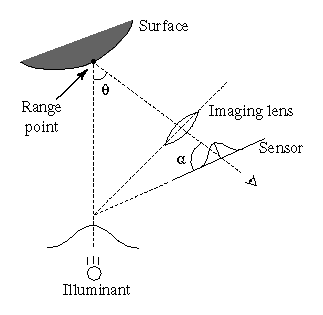Figure 1: Optical triangulation geometry. The angle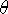is the triangulation angle while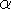is the tilt of the sensor plane needed to keep the laser plane in focus.

# 2 Error in triangulation systems

For optical triangulation systems, the accuracy of the range data depends on proper interpretation of imaged light reflections. The most common approach is to reduce the problem to one of finding the ``center'' of a one dimensional pulse, where the ``center'' refers to the position on the sensor which hopefully maps to the center of the illuminant. Typically, researchers have opted for a statistic such as mean, median or peak of the imaged light as representative of the center. These statistics give the correct answer when the surface is perfectly planar, but they are generally inaccurate whenever the surface perturbs the shape of the illuminant.

## 2.1 Geometric intuition

Perturbations of the shape of the imaged illuminant occur whenever:

• The surface reflectance varies.
• The surface geometry deviates from planarity.
• The light paths to the sensor are partially occluded.
• The surface is sufficiently rough to cause laser speckle.
In Figure 2, we give examples of how the first three circumstances result in range errors even for an ideal triangulation system with infinite sensor resolution and perfect calibration. For purposes of illustration, we omit the imaging optics of Figure 1 and treat the sensor as a one dimensional orthographic sensor. Further, we assume an illuminant of Gaussian cross-section, and we use the mean for determining the center of an imaged pulse. Figure 2a shows how a step reflectance discontinuity results in range points that do not lie on the surface. Figure 2b and 2c provide two examples of shape variations resulting in range errors. Note that in Figure 2c, the center of the illuminant is not even striking a surface. In this case, a measure of the center of the pulse results in a range value, when in fact the correct answer is to return no range value whatever. Finally, Figure 2d shows the effect of occluding the line of sight between the illuminated surface and the sensor. This range error is very similar to the error encountered in Figure 2c.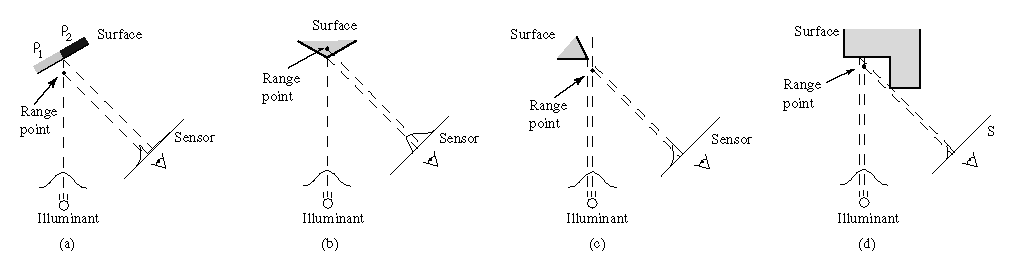Figure 2: Range errors using traditional triangulation methods. (a) Reflectance discontinuity. (b) Corner. (c) Shape discontinuity with respect to the illumination. (d) Sensor occlusion.

The fourth source of range error is laser speckle, which arises when coherent laser illumination bounces off of a surface that is rough compared to a wavelength . The surface roughness introduces random variations in optical path lengths, causing a random interference pattern throughout space and at the sensor. The result is an imaged pulse with a noise component that affects the mean pulse detection, causing range errors even from a planar target.

## 2.2 Quantifying the error

To quantify the errors inherent in using mean pulse analysis, we have computed the errors introduced by reflectance and shape variations for an ideal triangulation system with a single Gaussian illuminant. We take the beam width, w, to be the distance between the beam center and the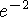point of the irradiance profile, a convention common to the optics literature. We present the range errors in a scale invariant form by dividing all distances by the beam width. Figure 3 illustrates the maximum deviation from planarity introduced by scanning reflectance discontinuities of varying step magnitudes for varying triangulation angles. As the size of the step increases, the error increases correspondingly. In addition, smaller triangulation angles, which are desirable for reducing the likelihood of missing data due to sensor occlusions, actually result in larger range errors. This result is not surprising, as sensor mean positions are converted to depths through a division by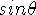, whereis the triangulation angle, so that errors in mean detection translate to larger range errors for smaller triangulation angles.

Figure 4 shows the effects of a corner on range error, where the error is taken to be the shortest distance between the computed range data and the exact corner point. The corner is oriented so that the illumination direction bisects the corner's angle as shown in Figure 2b. As we might expect, a sharper corner results in greater compression of the left side of the imaged Gaussian relative to the right side, pushing the mean further to the right on the sensor and pushing the triangulated point further behind the corner. In this case, the triangulation angle has little effect as the division byis offset almost exactly by the smaller observed left/right pulse compression imbalance.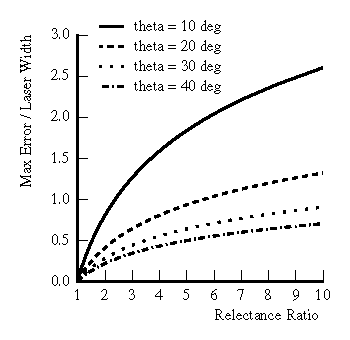Figure 3: Plot of errors due to reflectance discontinuities for varying triangulation angles (theta).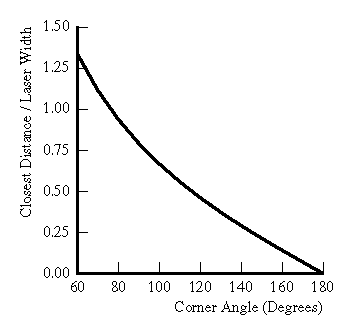Figure 4: Plot of errors due to corners.

One possible strategy for reducing these errors would be to decrease the width of the beam and increase the resolution of the sensor. However, diffraction limits prevent us from focusing the beam to an arbitrary width. The limits on focusing a Gaussian beam with spherical lenses are well known . In recent years, Bickel, et al,  have explored the use of axicons (e.g., glass cones and other surfaces of revolution) to attain tighter focus of a Gaussian beam. The refracted beam, however, has a zeroth order Bessel function cross-section; i.e., it has numerous side-lobes of non-negligible irradiance. The influence of these side-lobes is not well-documented and would seem to complicate triangulation.

# 3 A New Method: Spacetime Analysis

The previous section clearly demonstrates that analyzing each imaged pulse using a low order statistic leads to systematic range errors. We have found that these errors can be reduced or eliminated by analyzing the time evolution of the pulses.

## 3.1 Geometric intuition

Figure 5 illustrates the principle of spacetime analysis for a laser triangulation scanner with Gaussian illuminant and orthographic sensor as it translates across the edge of an object. As the scanner steps to the right, the sensor images a smaller and smaller portion of the laser cross-section. By time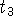, the sensor no longer images the center of the illuminant, and conventional methods of range estimation fail. However, if we look along the lines of sight from the corner to the laser and from the corner to the sensor, we see that the profile of the laser is being imaged over time onto the sensor (indicated by the dotted Gaussian envelope). Thus, we can find the coordinates of the corner point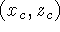by searching for the mean of a Gaussian along a constant line of sight through the sensor images. We can express the coordinates of this mean as a time and a position on the sensor, where the time is in general between sensor frames and the position is between sensor pixels. The position on the sensor indicates a depth, and the time indicates the lateral position of the center of the illuminant. In the example of Figure 5, we find that the spacetime Gaussian corresponding to the exact corner has its mean at position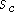on the sensor at a time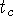between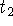andduring the scan. We extract the corner's depth by triangulating the center of the illuminant with the line of sight corresponding to the sensor coordinate, while the corner's horizontal position is proportional to the time.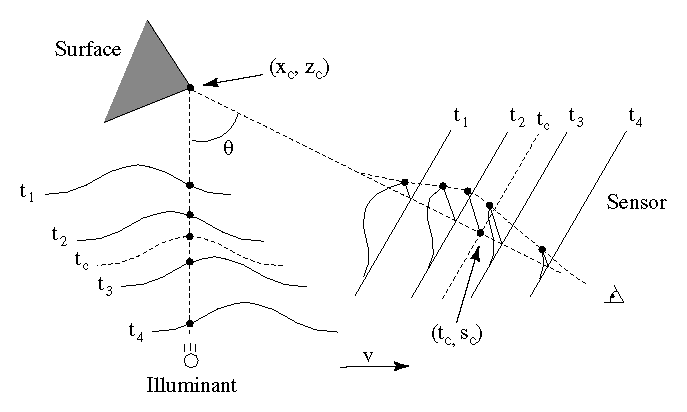Figure 5: Spacetime mapping of a Gaussian illuminant. As the light sweeps across the corner point, the sensor images the shape of the illuminant over time.

## 3.2 A complete derivation

For a more rigorous analysis, we consider the time evolution of the irradiance from a translating differential surface element,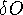, as recorded at the sensor. We refer the reader to Figure 6 for a description of coordinate systems; note that in contrast to the previous section, the surface element is translating instead of the illuminant-sensor assembly.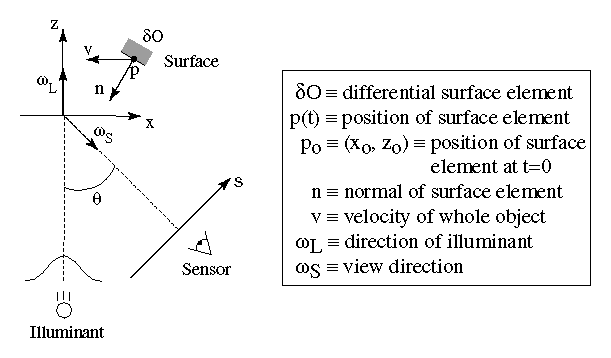Figure 6: Triangulation scanner coordinate system. A depiction of the coordinate systems and the vectors relevant to a moving differential element.

The element has a normal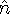and an initial position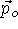and is translating with velocity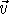, so that: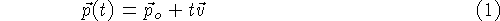Our objective is to compute the coordinates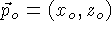given the temporal irradiance variations on the sensor. For simplicity, we assume that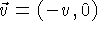. The illuminant we consider is a laser with a unidirectional Gaussian radiance profile. We can describe the total radiance reflected from the element to the sensor as: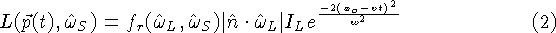where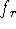is the bidirectional reflection distribution function (BRDF) of the point,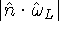is the cosine of the angle between the surface and illumination. The remaining terms describe a point moving in the x-direction under the Gaussian illuminant of width w and power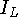.

Projecting the point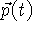onto the sensor, we find: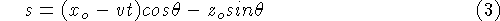where s is the position on the sensor andis the angle between the sensor and laser directions. We combine Equations 2-3 to give us an equation for the irradiance observed at the sensor as a function of time and position on the sensor: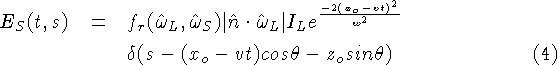To simplify this expression, we condense the light reflection terms into one measure: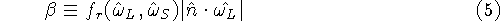which we will refer to as the reflectance coefficient of point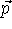for the given illumination and viewing directions. We also note that x=vt is a measure of the relative x-displacement of the point during a scan, and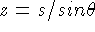is the relation between sensor coordinates and depth values along the center of the illuminant. Making these substitutions we have: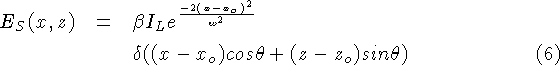This equation describes a Gaussian running along a tilted line through the spacetime sensor plane or ``spacetime image''. We define the ``spacetime image'' to be the image whose columns are filled with sensor scanlines that evolve over time. Through the substitutions above, position within a column of this image represents displacement in depth, and position within a row represents time or displacement in lateral position. Figure 7 shows the theoretical spacetime image of a single point based on the derivation above, while Figures 8a and  8b shows the spacetime image generated during a real scan. From Figure 7, we see that the tilt angle is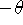with respect to the z-axis, and the width of the Gaussian along the line is: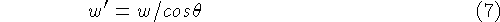The peak value of the Gaussian is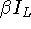, and its mean along the line is located at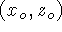, the exact location of the range point. Note that the angle of the line and the width of the Gaussian are solely determined by the fixed parameters of the scanner, not the position, orientation, or BRDF of the surface element.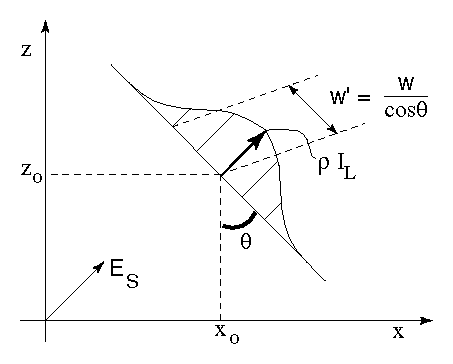Figure 7: Spacetime image of a point passing through a Gaussian illuminant.

Thus, extraction of range points should proceed by computing low order statistics along tilted lines through the sensor spacetime image, rather than along columns (scanlines) as in the conventional method. As a result, we can determine the position of the surface element independently of the orientation and BRDF of the element and independently of any other nearby surface elements. In theory, the decoupling of range determination from local shape and reflectance is complete. In practice, optical systems and sensors have filtering and sampling properties that limit the ability to resolve neighboring points. In Figure 8d, for instance, the extracted edges extend slightly beyond their actual bounds. We attribute this artifact to filtering which blurs the exact cutoffs of the edges into neighboring pixels in the spacetime image, causing us to find additional range values.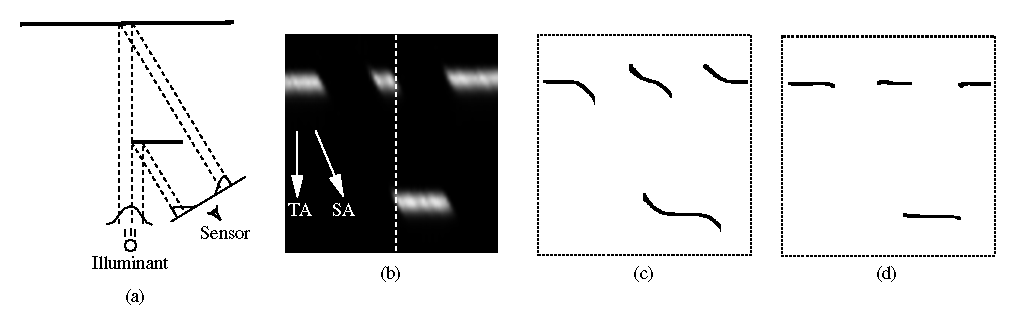Figure 8: From geometry to spacetime image to range data. (a) The original geometry. (b) The resulting spacetime image. TA indicates the direction of traditional analysis, while SA is the direction of the spacetime analysis. The dotted line corresponds to the scanline generated at the instant shown in (a). (c) Range data after traditional mean analysis. (d) Range data after spacetime analysis.

As a side effect of the spacetime analysis, the peak of the Gaussian yields the irradiance at the sensor due to the point. Thus, we automatically obtain an intensity image precisely registered to the range image.

## 3.3 Generalizing the geometry

We can easily generalize the previous results to other scanner geometries under the following conditions:

• The illuminant direction is constant over the path of each range point.
• The sensor is orthographic.
• The motion is purely translational.
These conditions ensure that the reflectance coefficient,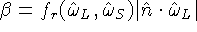, is constant. Note that the illumination need only be directional; coherent or incoherent light of any pattern is acceptable. Further, the translational motion need not be of constant speed, only constant direction; we can correct for known variations in speed by applying a suitable warp to the spacetime image.

We can weaken each of these restrictions if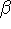does not vary appreciably for each point as it passes through the illuminant. A perspective sensor is suitable if the changes in viewing directions are relatively small for neighboring points inside the illuminant. This assumption of ``local orthography'' has yielded excellent results in practice. In addition, we can tolerate a rotational component to the motion as long as the radius of curvature of the point path is large relative to the beam width, again minimizing the effects on.

## 3.4 Correcting laser speckle

The discussion in sections 3.1-3.3 show how we can go about extracting accurate range data in the presence of shape and reflectance variations, as well as occlusions. But what about laser speckle? Empirical observation of the time evolution of the speckle pattern with our optical triangulation scanner strongly suggests that the image of laser speckle moves as the surface moves. The streaks in the spacetime image of Figure 8b correspond to speckle noise, for the object has uniform reflectance and should result in a spacetime image with uniform peak amplitudes. These streaks are tilted precisely along the direction of the spacetime analysis, indicating that the speckle noise adheres to the surface of the object and behaves as a noisy reflectance variation. Other researchers have observed a ``stationary speckle'' phenomenon as well . Proper analysis of this problem is an open question, likely to be resolved with the study of the governing equations of scalar diffraction theory for imaging of a rough translating surface under coherent Gaussian beam illumination .

# 4 Implementation

We have implemented the spacetime analysis presented in the previous section using a commercial laser triangulation scanner and a real-time digital video recorder.

## 4.1 Hardware

The optical triangulation system we use is a Cyberware MS platform scanner. This scanner collects range data by casting a laser stripe on the object and by observing reflections with a CCD camera positioned at an angle of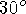with respect to the plane of the laser. The platform can either translate or rotate an object through the field of view of the triangulation optics. The laser width varies from 0.8 mm to 1.0 mm over the field of view which is approximately 30 cm in depth and 30 cm in height. Each CCD pixel images a portion of the laser plane roughly 0.5 mm by 0.5 mm. Although the Cyberware scanner performs a form of peak detection in real time, we require the actual video frames of the camera for our analysis. We capture these frames with an Abekas A20 video digitizer and an Abekas A60 digital video disk, a system that can acquire 486 by 720 size frames at 30 Hz. These captured frames have approximately the same resolution as the Cyberware range camera, though they represent a resampling of the reconstructed CCD output.

## 4.2 Algorithms

Using the principles of section 3, we can devise a procedure for extracting range data from spacetime images:

1. Perform the range scan and capture the spacetime images.
2. Rotate the spacetime images by.
3. Find the statistics of the Gaussians in the rotated coordinates.
4. Rotate the means back to the original coordinates.
In order to implement step 1 of this algorithm, we require a sequence of CCD images. Most commercial optical triangulation systems discard each CCD image after using it (e.g. to compute a stripe of the range map). As described in section 4.1, we have assembled the necessary hardware to record the CCD frames. In section 3, we discussed a one dimensional sensor scenario and indicated that perspective imaging could be treated as locally orthographic. For a two dimensional sensor, we can imagine the horizontal scanlines as separate one dimensional sensors with varying vertical (y) offsets. Each scanline generates a spacetime image, and by stacking the spacetime images one atop another, we define a spacetime volume. In general, we must perform our analysis along the paths of points, paths which may cross scanlines within the spacetime volume. However, we have observed for our system that the illuminant is sufficiently narrow and the perspective of the range camera sufficiently weak, that these paths essentially remain within scanlines. This observation allows us to perform our analysis on each spacetime image separately.

In step 2, we rotate the spacetime images so that Gaussians are vertically aligned. In a practical system with different sampling rates in x and z, the correct rotation angle can be computed as: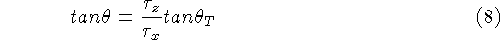whereis the new rotation angle,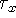and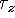are the sample spacing in x and z respectively, and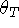is the triangulation angle. In order to determine the rotation angle,, for a given scanning rate and region of the field of view of our Cyberware scanner, we first determined the local triangulation angle and the sample spacings in depth (z) and lateral position (x). Equation 8 then yields the desired angle.

In step 3, we compute the statistics of the Gaussians along each rotated spacetime image raster. Our method of choice for computing these statistics is a least squares fit of a parabola to the log of the data. We have experimented with fitting the data directly to Gaussians using the Levenberg-Marquardt non-linear least squares algorithm , but the results have been substantially the same as the log-parabola fits. The Gaussian statistics consist of a mean, which corresponds to a range point, as well as a width and a peak amplitude, both of which indicate the reliability of the data. Widths that are far from the expected width and peak amplitudes near the noise floor of the sensor imply unreliable data which may be down-weighted or discarded during later processing (e.g., when combining multiple range meshes ). For the purposes of this paper, we discard unreliable data.

Finally, in step 4, we rotate the range points back into the global coordinate system.

Traditionally, researchers have extracted range data at sampling rates corresponding to one range point per sensor scanline per unit time. Interpolation of shape between range points has consisted of fitting primitives (e.g., linear interpolants like triangles) to the range points. Instead, we can regard the spacetime volume as the primary source of information we have about an object. After performing a real scan, we have a sampled representation of the spacetime volume, which we can then reconstruct to generate a continuous function. This function then acts as our range oracle, which we can query for range data at a sampling rate of our choosing. In practice, we can magnify the sampled spacetime volume prior to applying the range imaging steps described above. The result is a range grid with a higher sampling density based directly on the imaged light reflections.

# 5 Results

## 5.1 Reflectance correction

To evaluate the tolerance of the spacetime method to changes in reflectance, we performed two experiments, one quantitative and the other qualitative. For the first experiment, we generated planar cards with step reflectance changes varying from about 1:1 to 10:1 and scanned them at an angle of(roughly facing the sensor). Figure 9 shows a plot of maximum deviations from planarity when using traditional per scanline mean analysis and our spacetime analysis. The spacetime method has clearly improved over the old method, yielding up to 85% reductions in range errors.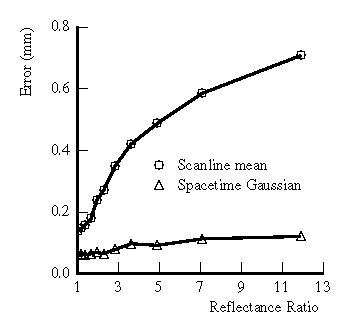Figure 9: Measured error due to varying reflectance steps.

For qualitative comparison, we produced a planar sheet with the word ``Reflectance'' printed on it. Figure 10 shows the results. The old method yields a surface with the characters well-embossed into the geometry, whereas the spacetime method yields a much more planar surface indicating successful decoupling of geometry and reflectance.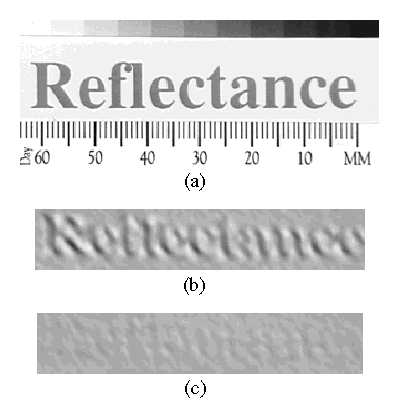Figure 10: Reflectance card. (a) Photograph of a planar card with the word ``Reflectance'' printed on it, and shaded renderings of the range data generated by (b) mean pulse analysis and (c) spacetime analysis.

## 5.2 Shape correction

We conducted several experiments to evaluate the effects of shape variation on range acquisition. In the first experiment, we generated corners of varying angles by abutting sharp edges of machined aluminum wedges which are painted white. Figure 11 shows the range errors that result for traditional and spacetime methods. Again, we see an increase in accuracy, though not as great as in the reflectance case.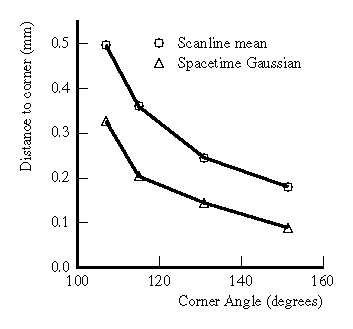Figure 11: Measured error due to corners of varying angles.

We also scanned two 4 mm strips of paper at an angle of(roughly facing the sensor) to examine the effects of depth continuity. Figure 12b shows the ``edge curl'' observed with the old method, while the spacetime method in Figure 12c shows a significant reduction of this artifact under spacetime analysis. We have found that the spacetime method reduces the length of the edge curl from an average of 1.1 mm to an average of approximately 0.35 mm.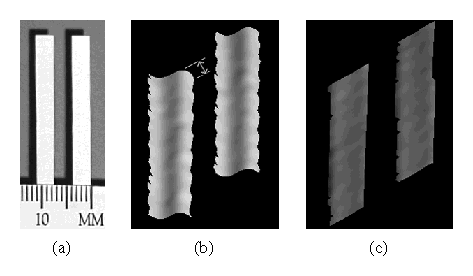Figure 12: Depth discontinuities and edge curl. (a) Photograph of two strips of paper, and shaded renderings of the range data generated by (b) mean pulse analysis and (c) spacetime analysis. The ``edge curl'' indicated by the hash-marks in (b) is 1.1mm.

Finally, we impressed the word ``shape'' onto a plastic ribbon using a commonly available label maker. In Figure 10, we wanted the word ``Reflectance'' to disappear because it represented changes in reflectance rather than in geometry. In Figure 13, we want the word ``Shape'' to stay because it represents real geometry. Furthermore, we wish to resolve it as highly as possible. Figure 13 shows the result. Using the scanline mean method, the word is barely visible. Using the new spacetime analysis, the word becomes legible.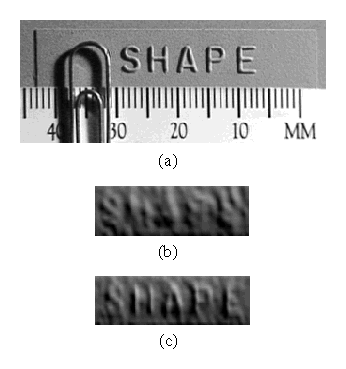Figure 13: Shape ribbon. (a) Photograph of a surface with raised lettering (letters are approx. 0.3 mm high), and renderings of the range data generated by (b) mean pulse analysis and (c) spacetime analysis.

## 5.3 Speckle reduction

We performed range scans on the planar surfaces and generated range points using the traditional and spacetime methods. After fitting planes to range points, we found a 30-60% reduction in average deviation from planarity when using the spacetime analysis.

## 5.4 A complex object

Figure 14 shows the results of scanning a model tractor. Figure 14b is a rendering of the data generated by the Cyberware scanner hardware and is particularly noisy. This added noisiness results from the method of pulse analysis performed by the hardware, a method similar to peak detection. Peak detection is especially susceptible to speckle noise, because it extracts a range point based on a single value or small neighborhood of values on a noisy curve. Mean analysis tends to average out the speckle noise, resulting in smoother range data as shown in Figure 14c. Figure 14d shows our spacetime results and Figure 14e shows the spacetime results with 3X interpolation and resampling of the spacetime volume as described in section 4.2. Note the sharper definition of features on the body of the tractor and less jagged edges in regions of depth discontinuity.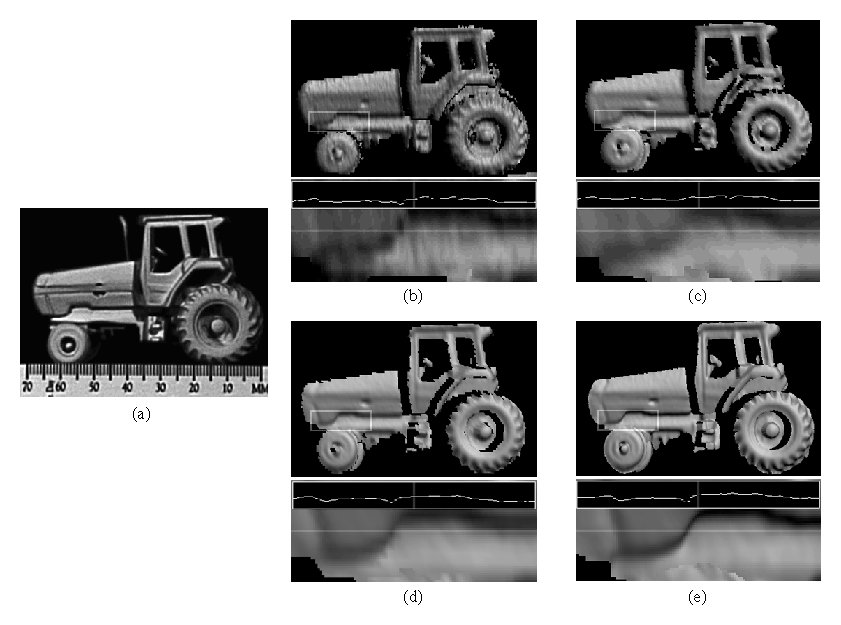Figure 14: Model tractor. (a) Photograph of original model and shaded renderings of range data generated by (b) the Cyberware scanner hardware, (c) mean pulse analysis, (d) our spacetime analysis, and (e) the spacetime analysis with 3X interpolation of the spacetime volume before fitting the Gaussians. Below each of the renderings is a blow-up of one section of the tractor body (indicated by rectangle on rendering) with a plot of one row of pixel intensities.

## 5.5 Remaining sources of error

The results we presented in this section clearly show that the spacetime analysis yields more accurate range data, but the results are imperfect due to system limitations. These limitations include:

• CCD noise
• Finite sensor resolution
• Optical blurring and electronic filtering
• Quantization errors
• Calibration errors
• Surface-surface inter-reflections
In addition, we observed some electronic artifacts in our Cyberware scanner that influenced our results. We expect, however, that any measures taken to reduce the effects of the limiting factors described above will lead to higher accuracy. By contrast, if one uses traditional methods of range extraction, then increasing sensor resolution and reducing the effects of filtering alone will not significantly increase tolerance to reflectance and shape changes when applying the traditional methods of range extraction.

# 6 Conclusion

We have described several of the systematic limitations in traditional methods of range acquisition with optical triangulation range scanners, including intolerance to reflectance and shape changes and speckle noise. By analyzing the time evolution of the reflected light imaged onto the sensor, we have shown that distortions induced by shape and reflectance changes can be corrected, while the influence of laser speckle can be reduced. In practice, we have demonstrated that we can significantly reduce range distortions with existing hardware. Although the spacetime method does not completely eliminate range artifacts in practice, it has proven to reduce the artifacts in all experiments we have conducted.

In future work, we plan to incorporate the improved range data with algorithms that integrate partial triangulation scans into complete, unified meshes. We expect this improved data to ease the process of estimating topology, especially in areas of high curvature which are prone to edge curl artifacts. We will also investigate methods for increasing the resolution of the existing hardware by registering and deblurring multiple spacetime images . Finally, we hope to apply the results of scalar diffraction theory to put the achievement of speckle reduction on sound theoretical footing.

## Acknowledgments

We thank the people of Cyberware for the use of the range scanner and for their help in accessing the raw video output from the range camera.

## References

1
R. Baribeau and M. Rioux. Influence of speckle on laser range finders. Applied Optics, 30(20):2873-2878, July 1991.

2
Paul Besl. Advances in Machine Vision, chapter 1 - Active optical range imaging sensors, pages 1-63. Springer-Verlag, 1989.

3
G. Bickel, G. Haulser, and M. Maul. Triangulation with expanded range of depth. Optical Engineering, 24(6):975-977, December 1985.

4
M. Buzinski, A. Levine, and W.H. Stevenson. Performance characteristics of range sensors utilizing optical triangulation. In Proceedings of the IEEE 1992 National Aerospace and Electronics Conference, NAECON 1992, pages 1230-1236, May 1992.

5
R.G. Dorsch, G. Hausler, and J.M. Herrmann. Laser triangulation: fundamental uncertainty in distance measurement. Applied Optics, 33(7):1306-1314, March 1994.

6
Joseph W. Goodman. Introduction to Fourier optics. McGraw-Hill, 1968.

7
J.W. Goodman. Laser Speckle and Related Phenomena, chapter 1 - Statistical properties of laser speckle patterns, pages 9-76. Springer-Verlag, 1984.

8
G. Hausler and W. Heckel. Light sectioning with large depth and high resolution. Applied Optics, 27(24):5165-5169, Dec 1988.

9
M. Irani and S. Peleg. Improving resolution by image registration. CVGIP: Graphical Models and Image Processing, 53(3):231-239, May 1991.

10
R.A. Jarvis. A perspective on range-finding techniques for computer vision. IEEE Trans. Pattern Analysis Mach. Intell., 5:122-139, March 1983.

11
T Kanade, A Gruss, and L Carley. A very fast vlsi rangefinder. In 1991 IEEE International Conference on Robotics and Automation, volume 39, pages 1322-1329, April 1991.

12
J.L. Mundy and G.B. Porter. Three-dimensional machine vision, chapter 1 - A three-dimensional sensor based on structured light, pages 3-61. Kluver Academic Publishers, 1987.

13
William H. Press, Brian P. Flannery, Saul A. Teukolsky, and William T. Vetterling. Numerical Recipes in C. Cambridge University Press, 1986.

14
M. Rioux, G. Bechthold, D. Taylor, and M. Duggan. Design of a large depth of view three-dimensional camera for robot vision. Optical Engineering, 26(12):1245-1250, Dec 1987.

15
A.E. Siegman. Lasers. University Science Books, 1986.

16
M. Soucy, D. Laurendeau, D. Poussart, and F. Auclair. Behaviour of the center of gravity of a reflected gaussian laser spot near a surface reflectance discontinuity. Industrial Metrology, 1(3):261-274, Sept 1990.

17
T. Strand. Optical three dimensional sensing. Optical Engineering, 24(1):33-40, Jan-Feb 1983.

18
G. Turk and M. Levoy. Zippered polygon meshes from range images. In SIGGRAPH 94 Conference Proceedings, pages 311-318, July 1994.

# About this document ...

Better Optical Triangulation through Spacetime Analysis

This document was generated using the LaTeX2HTML translator Version 96.1 (Feb 5, 1996) Copyright © 1993, 1994, 1995, 1996, Nikos Drakos, Computer Based Learning Unit, University of Leeds.

The command line arguments were:
latex2html -dir /usr/tmp/st -show_section_numbers -address curless@graphics.stanford.edu -split 0 paper.tex.

The translation was initiated by Brian Curless on Sun Sep 8 11:41:40 PDT 1996

curless@graphics.stanford.edu